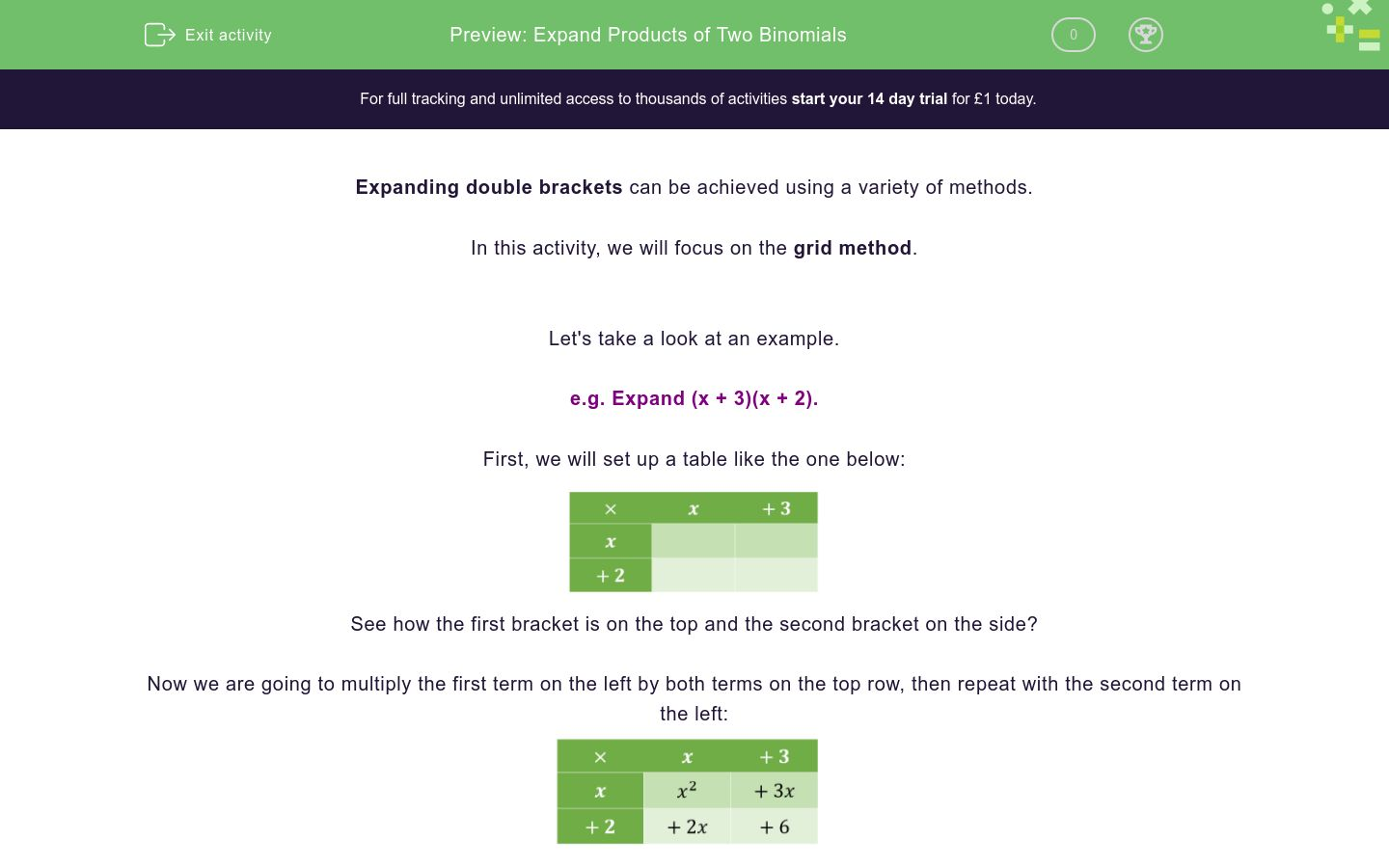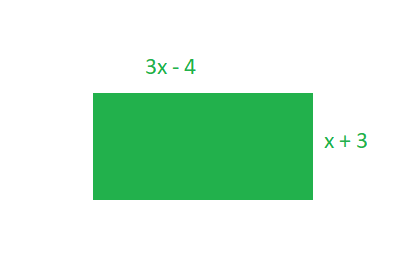# Expand Products of Two Binomials

In this worksheet, students will use the grid method to expand double brackets then simplify answers.Key stage:  KS 4

GCSE Subjects:   Maths

GCSE Boards:   Pearson Edexcel, OCR, Eduqas, AQA

Curriculum topic:   Algebra

Curriculum subtopic:   Notation, Vocabulary and Manipulation, Algebraic Expressions

Difficulty level:### QUESTION 1 of 10

Expanding double brackets can be achieved using a variety of methods.

In this activity, we will focus on the grid method.

Let's take a look at an example.

e.g. Expand (x + 3)(x + 2).

First, we will set up a table like the one below:See how the first bracket is on the top and the second bracket on the side?

Now we are going to multiply the first term on the left by both terms on the top row, then repeat with the second term on the left:We need to make sure that our signs are correct here.

A positive number multiplied by another positive gives a positive outcome.

A negative number multiplied by another negative also gives a positive outcome.

But a negative number multiplied by a positive always gives a negative outcome.

Now take all the terms we've just placed in a table and simplify as much as possible:

x² + 3x + 2x + 6 = x² + 5x + 6

So in summary, (x + 3)(x + 2) = x² + 5x + 6

In this activity, we will practise expanding double brackets using the grid method described above and then simplifying our answers.

You may want to have a pen and paper to hand so that you can draw out your own grids.

Here is a grid created for expanding (x + 5)(x + 3):What is the simplified answer to (x + 5)(x + 3)?

26x3

x2 + 3x + 5x + 15

x2 + 8x + 15

x2 + 15x + 15

What is the missing element (represented by ?) in the grid below?- 11

+ 11

- 30

+ 30

What is the missing element (represented by ?) in the grid below?+ 12

+ 3

- 3

- 12

Use the grid method to expand and simplify:

(x + 2)(x - 5)x2 + 3x - 10

x2 - 3x + 10

x2 + 3x + 10

x2 - 3x - 10

Match each bracket pair below with its correct simplified expansion.## Column B

(2x + 5)(x + 3)
2x2 + 11x + 15
(2x - 5)(x + 3)
2x2 - x - 15
(2x - 5)(x - 3)
2x2 - 11x + 15
(2x + 5)(x - 3)
2x2 + x - 15

Pick the other bracket that can be expanded with (2x + 1) to give:

2x² + 3x + 1

 (2x - 1) (x + 1) (x + 3) (x - 3) (2x + 1)

Which expression below represents the area of this rectangle?3x2 + 5x - 12

3x2 - 5x - 12

3x2 + 5x + 12

3x2 - 5x + 12

Match each bracket pair below with its correct simplified expansion.## Column B

(5x - 6)(2x + 3)
10x2 + 3x - 18
(5x + 6)(2x + 3)
10x2 - 27x + 18
(5x - 6)(2x - 3)
10x2 - 3x - 18
(5x + 6)(2x - 3)
10x2 + 27x + 18

Expand and simplify:

(5x + 4)²

25x2 + 16

25x2 + 4

25x2 + 8x + 16

25x2 + 40x + 16

Your friend has expanded (4x + 1)(x - 5) by creating the grid below.They have then written these products out below in preparation for simplifying to find their final answer.

However, they have made an error.

Underline the element of the expression below which is incorrect.

4x² + 20x + x - 5
• Question 1

Here is a grid created for expanding (x + 5)(x + 3):What is the simplified answer to (x + 5)(x + 3)?

x2 + 8x + 15
EDDIE SAYS
Once we have the grid filled out, we need to recombine and then simplify the terms. Let's start by listing all the terms we see in the grid: x2 + 5x + 3x + 15 Which of these elements can be simplified? We can add the two terms involving x: 5x + 3x = 8x No other terms can be simplified further, as we cannot see any other common factors. So our simplest possible answer is: x2 + 8x + 15
• Question 2

What is the missing element (represented by ?) in the grid below?+ 30
EDDIE SAYS
Remember that we need to multiply out each of the terms to fill in the grid squares. The missing value is in the square where - 6 and - 5 need to be multiplied. - 6 x - 5 = 30 Did you remember that the product of two negative numbers is always positive?
• Question 3

What is the missing element (represented by ?) in the grid below?+ 3
EDDIE SAYS
Here the ? is in the title of the right-hand column. So we need to work out what term can be multiplied with the values in the left-hand column to give the products we see in the grid. x × ? = 3x -6 × ? = -18 So the value of the ? must be 3 for both the expressions above to be true. Check this answer works by adding it to the title and wiping the values in the grid, then multiply to check you reach the same products.
• Question 4

Use the grid method to expand and simplify:

(x + 2)(x - 5)x2 - 3x - 10
EDDIE SAYS
Expanding (x + 2)(x - 5) should give you the products: x², 2x, -5x, -10. Then we need to put these elements back together and simplify: x² + 2x - 5x - 10 We can simplify the x terms, so our final answer is: x² - 3x - 10
• Question 5

Match each bracket pair below with its correct simplified expansion.## Column B

(2x + 5)(x + 3)
2x2 + 11x + 15
(2x - 5)(x + 3)
2x2 + x - 15
(2x - 5)(x - 3)
2x2 - 11x + 15
(2x + 5)(x - 3)
2x2 - x - 15
EDDIE SAYS
Did you use the grid in each case? Put the terms of the first brackets in the top row and the terms of the second bracket down the left-hand side. The difficult factor here is the different signs. Be very careful how you multiply out the terms. Remember: positive × positive = positive outcome positive × negative = negative outcome negative × positive = negative outcome negative × negative = positive outcome Let's work through the first match together. (2x + 5)(x + 3) = 2x2 + 6x + 5x + 15 We can simplify the x terms to reach an answer of: 2x2 + 11x + 15 Can you match the other pairs independently using this example and a grid of your own to support you?
• Question 6

Pick the other bracket that can be expanded with (2x + 1) to give:

2x² + 3x + 1

 (2x - 1) (x + 1) (x + 3) (x - 3) (2x + 1)
EDDIE SAYS
A good way to think about this question is that we know the values in the left-hand column of our grid and we know all the products in the squares, but we do not know the values in the top row. Let's call our missing values ? and ??. We know that: 2x × ? = 2x2 and 1 × ?? = 1 So ? = x and ?? = 1 Which matches to (x + 1) in the options. We can check this is correct by completing the grid using these brackets and checking we reach the answer of 2x2 + 3x + 1.
• Question 7

Which expression below represents the area of this rectangle?3x2 + 5x - 12
EDDIE SAYS
How do we find the area of a rectangle? Length x width Here the length is (3x - 4) and the width is (x + 3), so the area of this rectangle can be expressed as: (3x - 4)(x + 3) Using the grid method to expand the brackets, we find that: 3x² - 4x + 9x - 12 = 3x² + 5x - 12
• Question 8

Match each bracket pair below with its correct simplified expansion.## Column B

(5x - 6)(2x + 3)
10x2 + 3x - 18
(5x + 6)(2x + 3)
10x2 + 27x + 18
(5x - 6)(2x - 3)
10x2 - 27x + 18
(5x + 6)(2x - 3)
10x2 - 3x - 18
EDDIE SAYS
These look all very similar, don't they? Make sure you get the signs right here; they make all the difference! When you place numbers in the grid, always place them together with a sign immediately before them. Let's work through one of these together. (5x - 6)(2x - 3) = 10x2 - 12x - 15x + 18 = 10x2 - 27x + 18 Did you remember that two negative multipliers give a positive outcome, but a negative and a positive give a negative outcome? Work through the other matches independently using a grid and this example to help you.
• Question 9

Expand and simplify:

(5x + 4)²

25x2 + 40x + 16
EDDIE SAYS
Did you spot that this question is actually about expanding double brackets? Squaring means multiplying by itself, so (5x + 4)² means (5x + 4)(5x + 4). If we multiply these two brackets in a grid, we reach: 25x² + 20x + 20x + 16 We can then simplify by grouping the x terms to get an answer of: 25x² + 40x + 16
• Question 10

Your friend has expanded (4x + 1)(x - 5) by creating the grid below.They have then written these products out below in preparation for simplifying to find their final answer.

However, they have made an error.

Underline the element of the expression below which is incorrect.

4x² + 20x + x - 5
EDDIE SAYS
They have copied each number and sign from their grid correctly, so an error has not occurred here. Let's check that their calculations then: 4x × x = 4x2 so this is correct 4x × -5 = -20x which is not correct 1 × x = x which is correct 1 x -5 = -5 which is also correct So the sign in front of 20x is incorrect. The correct answer should read: 4x² - 19x - 5 not 4x² + 21x - 5 which would have been your friend's answer. Great work if you spotted that sneaky sign swap to complete this activity!
---- OR ----

Sign up for a £1 trial so you can track and measure your child's progress on this activity.

### What is EdPlace?

We're your National Curriculum aligned online education content provider helping each child succeed in English, maths and science from year 1 to GCSE. With an EdPlace account you’ll be able to track and measure progress, helping each child achieve their best. We build confidence and attainment by personalising each child’s learning at a level that suits them.

Get started